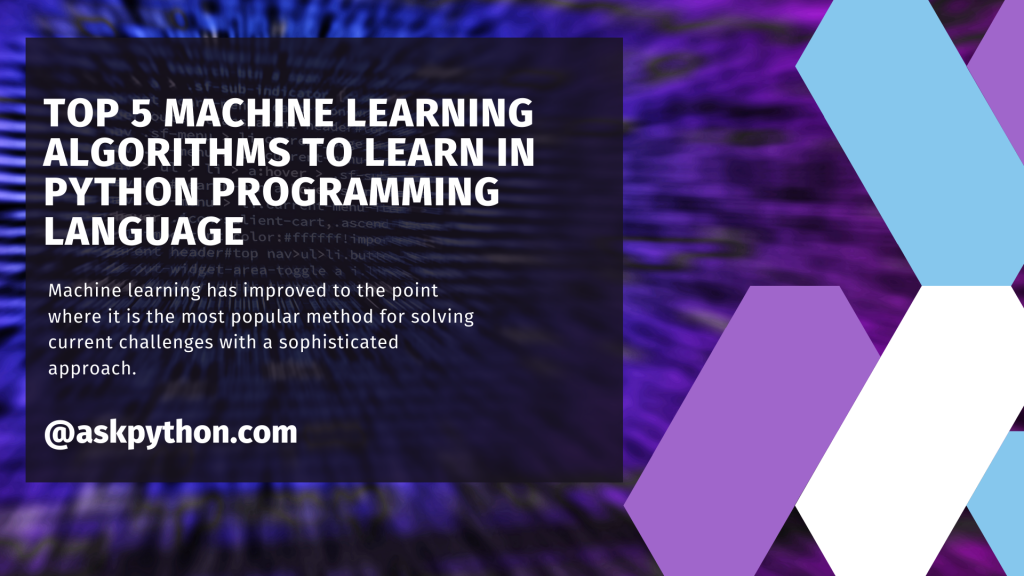# Top 5 Machine Learning Algorithms In Python You Must KnowHere are the top five machine learning algorithms for beginners. Machine learning has improved to the point where it is the most popular method for solving current challenges with a sophisticated approach.

## Algorithm 1: Neural Network

One of our finest achievements is an artificial neural network. As illustrated in the illustration, we have developed a network of nodes that are interconnected to resemble the neurons in our brain.

In layman’s words, each neuron receives information from another neuron, processes it, and sends it to another neuron as output.

Each circular node represents an artificial neuron, and each arrow symbolizes a link between the output of one neuron and the input of another.

Instead of predicting a purchase or sell decision, neural networks can be more effective when used to discover interdependencies across different asset classes.

## Algorithm 2: Naive Bayes

The Naive Bayes classifier is a well-known probability classifier that can discriminate between numerous items.

It is well-known for its real-time categorization because of its speed and precision. The Bayes theorem primarily determines the likelihood of an event occurring.

It is simple and quick to anticipate the kind of test data set. It also excels in multi-class prediction.

Because of its superior mathematical approach, the Naive Bayes model is simple to implement and especially helpful for very large data sets.

Because of its capacity to categorize in real-time, this method outperforms another algorithm.

## Algorithm 3: K-means Clustering

The purpose of these Machine Learning Algorithms is to label data points based on their similarity. As a result, we do not define the clusters before running the method; rather, the algorithm discovers these clusters as it runs.

K-means clustering might be advantageous to traders who believe that there may be commonalities between various assets that are not visible on the surface.

## Algorithm 4: Logistic Regression

For binary classification, logistic regression is best suited. Logistic Regression is a function of f(x)= 1/(1+ex). This function is a decaying exponential function with a maximum value of 1.

The basic goal of the regression is to identify the best coefficients. We may use these coefficients to lessen the inaccuracy in our results.

## Algorithm 5: Principal Component Analysis

By decreasing variables, Principal Component Analysis (PCA) is used to make data easier to analyze and display.

Capturing the largest variance of the data in a new coordinate system with axes known as ‘principal components.’ Each orthogonal component is a linear combination of the original variables.

The presence of orthogonality between members means that there is no correlation between these components.

The first principal component captures the greatest amount of variation in the data. The second principal component represents the remaining variation in the data but contains variables that are unrelated to the first.

Similarly, all subsequent principal components capture the remaining variation while staying unrelated to the prior component.

## Conclusion

Congratulations! You just learned about the top 5 Machine Learning Algos to learn in the Python programming language. Hope you enjoyed it! 😇

Thank you for taking your time out! Hope you learned something new!! 😄